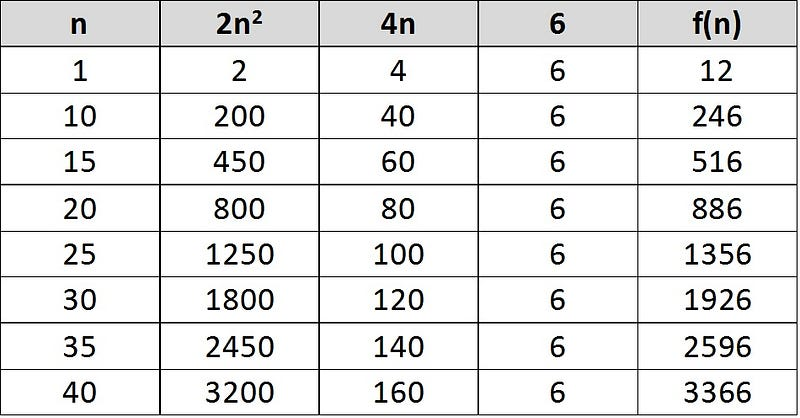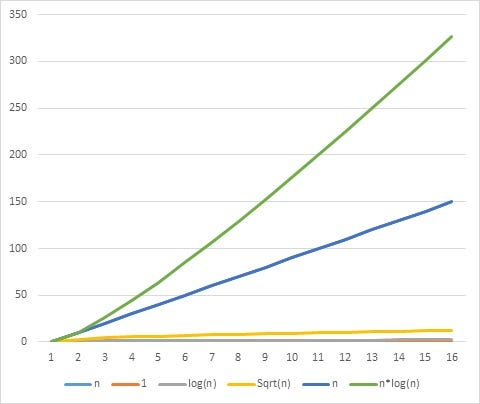## 1. Brief

You can always find O(n), O(log n) over the internet when people talking about the efficiency of the codes. Like any other magic which you will encounter, it will give you a big question mark. I assemble all the information I have, from my lectures, book readings, online information, personal understanding, then write down this article. Medium measures you can finish this article in 6 minutes, here are what you will get after the precious 6 minutes:

• How do we get the answer of Big O academically? (Rarely see online)
• How to measure the Big O for an algorithm practically?

A big plus: You don’t need any background to read this! Even a very beginner can understand this. Just a very little math (no computation). Hope it could help. Let’s start!

## 2. What is Big O?

It is a way to determine the efficiency of a program, or more specific, an algorithm. In most cases, it is about how efficient the process time is. But you should know that algorithm analysis is a fundamental computer science topic, algorithm analysis does not only involve time efficiency, it involves space(memory) efficiency as well. But Big O is all about the time efficiency in most cases (it can represent the space complexity).

## 3. The growth function:

The most frustrating problem I have faced when I first learn Big O notation is that how can people judge some complex algorithm using such simple notation. What is the black magic? The answer is “the growth function”.

Every algorithm is coded for solving problems. Let’s say there is an algorithm to sort an array. when we do efficiency analysis, we need to know how well this algorithm can perform. We need to know 2 factors:

• The size of the problem: it is the size of the array here.
• The key process which will influence the result time: Here is the number of comparisons we need to do. The more comparisons, the slower the algorithm will be.

That is to say, the algorithm’s efficiency can be defined in terms of the problem size and the processing step. A growth function shows the relationship between the two. It shows the time or space complexity relative to the problem size.

Before start the next section, Let’s assume the growth function for the sorting algorithm is like below:

f(n)=2n^2+4n+6

## 4. The asymptotic complexity

But we can’t use this kind of way to measure every algorithm, so, instead of knowing the accurate efficiency, we just need to know the “asymptotic complexity”, which is defined by the dominant term of a growth function. A dominant term is a term which increases most quickly as the size of problem increases. For the above growth function, let’s draw a table:It is very easy to find out that as n grows, the term 2n^2 dominates the result of the function, which is f(n). So, in this case, 2n^2 is our dominant term. Something important here, Saying that a term is a dominant term as n gets large does not mean that it is larger than the other terms for all values of n. You can see, when n=1, 4n and the constant (6) are both greater than 2n^2.

As the above paragraph, we can form a very rough equation:

Big O = Asymptotic Complexity = Dominant Term

Can we say the asymptotic complexity for the above formula is O(2n^2)? In fact, you can, but it is good for you to know that asymptotic complexity is also called “the order of the algorithm”. The word “order” here means “approximately”. And since we only have interested in the dominant term, that means we can ignore other terms and constants. So the constant 2 here can be eliminated, in fact, when we deal with some unknown numbers, 2n^2 and n^2 is nearly the same, right? So, we finally get to see something familiar:

O(n^2)

Now you know why the Wikipedia says: Big O notation is a mathematical notation.

## 5. Why I need Big O?

Some people may argue that we don’t need to know the Big O. Nowadays, the CPU and memory are both have strong parameters. Some algorithm which is slow today may become faster tomorrow due to the hardware upgrade. With the above table, you can know that the huge gap between the growth rate of O(2n^2)=O(n^2) and O(4n)=O(n). You should keep this in mind:

An algorithm can ugly enough to slow down the whole system and it is much easier for you to find a more efficient algorithm than to find a faster CPU.

## 6. How to measure the codes using Big O?

After understanding thing what’s really under the hood. Now let’s see some rules you can apply when analyzing. ( To be honest, Most of them are summarized by my programming teacher Micheal Albert xD ).

1. assignment statements and if statements that are only executed once regardless of the size of the problem are O(1).
2. a simple “for” loop from 0 to n (with no internal loops), contributes O(n) (linear complexity);
3. a nested loop of the same type (or bounded by the first loop parameter), gives O(n^2) (quadratic complexity);
4. a loop in which the controlling parameter is divided by two at each step (and which terminates when it reaches 1), gives O(log n) (logarithmic complexity);
5. a “while” loop may vary depends on the actual numbers of iterations it will run ( OK. It means as same as the for loop -_-. That is to say, for all kinds of loops, we only care about the actual number of iterations that they’ve executed before they hit the upper bond).
6. A loop with a not-O(1) execution inside, simply multiplies the complexity of the body of the loop, by the number of times the loop will execute.
7. When dealing with multiple statements, add them up.

You should be warned that some declarations may include initializations and some of these may be complex enough to factor into the efficiency of an algorithm.

## 7. One example to rule them all

Let’s take some Java code for example (finally, a coder can contribute something xD)

We can know from rule No.2 that it is O(n) for the for loop, but in order to know the Big O for the whole block, we need to know what does the `sumFromZero()` method do.

Using the above rule, we can know the overall time complexity for this method is:

• The assign statement: O(1);
• The for loop: O(n)
• The inside accumulation: O(1) so omit it;
• The println line is of course O(1);

Thus the overall complexity is O(n) for the `sumFromZero()` method.

In fact, with the growth function knowledge, you can write down the growth function for it to elaborate this process:

• f(n)=1+n+1 (Rule 6 and 7)
• Focus the dominant term only, ignore the others.
• So, O(n) finally

At last, for the first for loop, we get an O(n) inside an O(n). How to compute? We can simply apply rule 6. Now we get the answer: O(n^2). How excited! Just that simple.

## 8. The fast one and slow one:

Now we know how to analysis, we should know which one is faster. I will give you the order, from the fastest to slowest.

O(1) < O(log n) < O( Sqrt(n) ) < O(n) < O(n log n) < O(n^2) < O(n^3) < O(2^n)

I will show you a picture so you can understand the huge differences among these complexities.## 9. Some extension on the bounds

Let’s start from our beloved function:

f(n)=2n^2+4n+6

We know its time complexity is O(n^2), further, it means there is a constant m and some value of n, such that f(n)<=m*n^2 for all n > initial n, just like the above graph, as n increases, the f(n) starts to increase as well, but it will always less than the O(n^2).

that is to say, the asymptotic complexity, which is the order of the algorithm, it provides an upper bound to its growth function.

Other important notations:

• omega (Ω) which refers to a function that provides a lower bound
• theta (Θ) which refers to a function that provides both an upper and lower bound.

And of course, there is also a Little-o notation which is common in mathematics but rarer in computer science.

## 10. End of story

Now I hope you have sufficient knowledge to determine the Big O of an actual algorithm.
I will write more blogs relates to computer science and programming, in this “step by step with all the background” way.